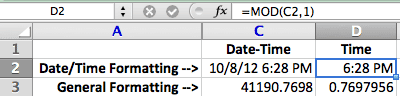# Extract Time with the MOD Function in Excel

I had a reader comment on my last post about how to extract time from a date-time number using the MOD function. Simple really.

The syntax is MOD(number,divisor). The MOD function returns the remainder after number is divided by divisor. A simple example is MOD(5,2), which equals one (1). It works like this: five (5) divided by two (2) equals two (2), with one (1) left over.

All numbers are evenly divisible by one (1) so the MOD function returns any fractional part when the second argument is one (1).

In the screen shot below, cell C2 has the Date-Time number: 10/8/12 6:28 PM. It has an underlying serial number: 41990.7698, which you can see in cell C3 with General formatting.Using the formula =MOD(C2,1) you can see the result in cells D2 and D3, with different cell formatting. Extracting the Time value from a Date-Time value is simple with the MOD function.## 2 thoughts on “Extract Time with the MOD Function in Excel”

1.Laura

Gregory, I have a fairly simple problem, but the solution eludes me.
I want to calculate total hours worked over a period of time, such as a week.
I can enter Start time, End time and subtract to get the hours for each day (all in Time format). No problem.
However, when I try to sum the hours for the days, I get an inaccurate result.
I tried converting the hours for each day from Time to General format in a separate column and then summing that column, but the results are still incorrect.

Briefly, this is what I’m looking for:
Start Stop Hours
08:00 17:00 9:00
08:00 16:35 8:35

Total Hours: 17:35

2.Laura

Please disregard my previous question. I realized that Excel was displaying the correct results…in 24-hour days. By changing the cell format to “[h]:mm”, I was able to see the answer in total hours.